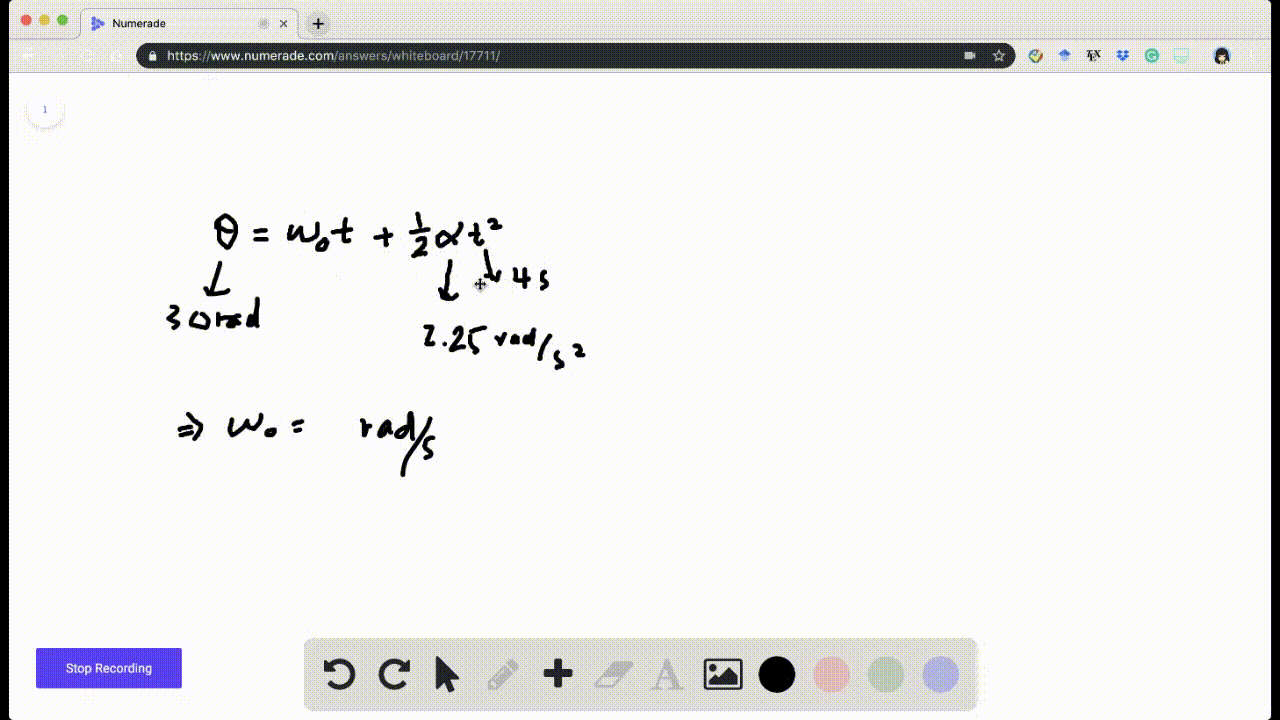Enroll in one of our FREE online STEM summer camps. Space is limited so join now!View Summer Courses## Discussion

You must be signed in to discuss.

## Video Transcript

So here it wants us to drop drive an equation for angular acceleration without solving for time So we can say our initial angular velocity will be twelve cleanser Radiance for seconds, our final angular velocity is going to be sixteen radiance per seconds. And then our change and angular displacement will be seven radiance. So we can first start off by saying omega half equals When they go, I plus out the tea and then we can say angular displacement Eagle singular initial in your basement, plus on mayor initial T plus half offa t squared and the fate of final minus data initial. It's something an equal Delta theta. And then here we can square we're going to square this term. So if we squared this term, this equation rather we would have Omega Final Squared equals Omega initial squared plus Alfa squared Times Square plus two off a national Omega initial Alfa team. So this would that would be this equation squared. And then what we're seeing here is that we can actually factor out a two Alfa so we can say Omega final squared equals omega initials there. Plus, we can say to Alfa, we could leave us with one over it. Two Alfa T squared plus omega initial T, and this is going to equal this So we can say that Omega initial T may serve no Omega initial squared, plus two Alfa times Delta A Fada will equal our final omega squared. And at this point, we have eliminated time so we can just solve for the angular acceleration and say that Alfa equals Omega Final squared minus omega initial squared, divided by two times Delta Theta. This is going to be sixteen squared, minus twelve squared call over two times seven radiance and the soon to be plus eight radiance per second squared. So this will be our angular acceleration without solving for time. And that's the end of the solution. Thank you for watching.